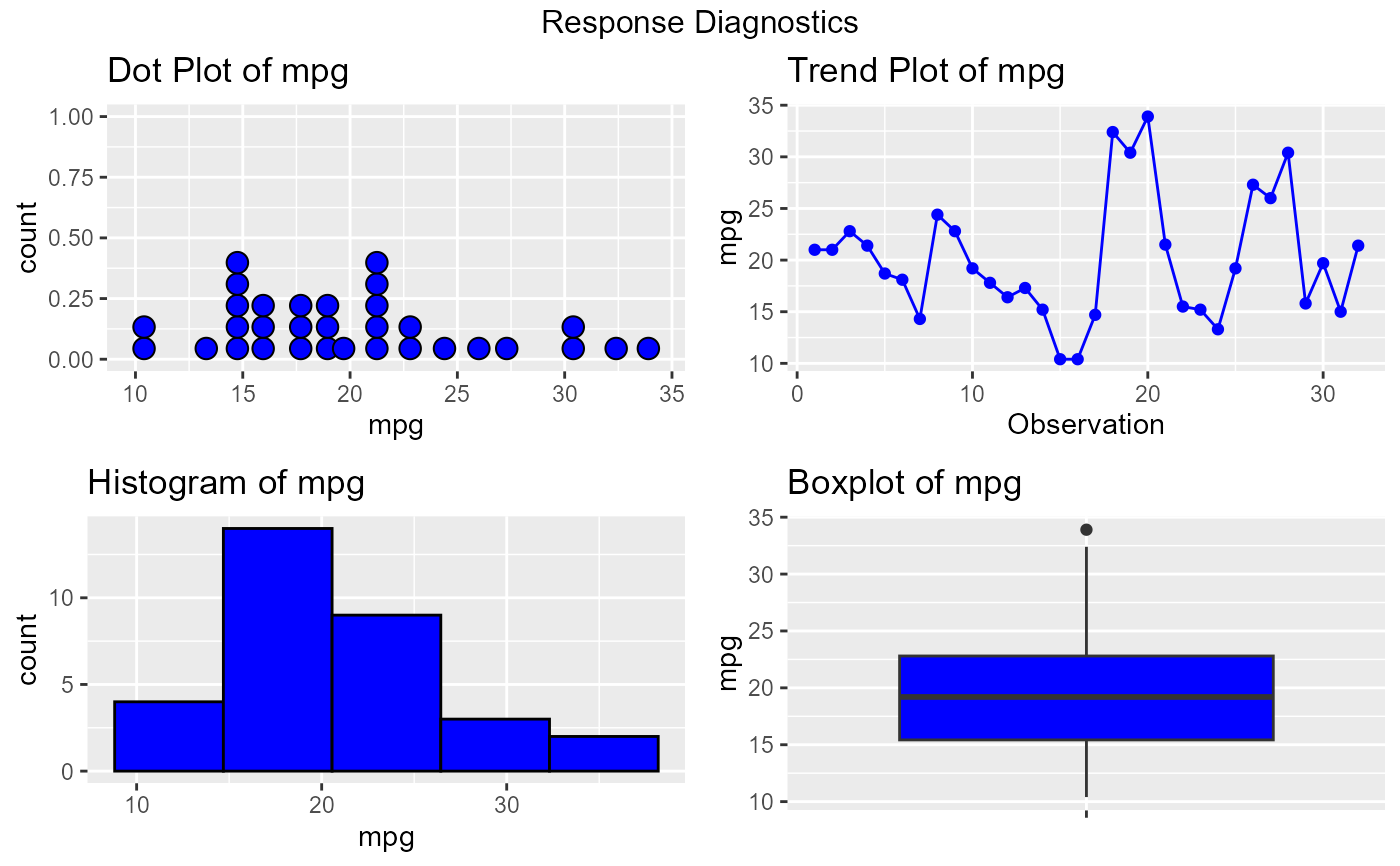Panel of plots to explore and visualize the response variable.

ols_plot_response(model, print_plot = TRUE)

## Arguments

model An object of class lm. logical; if TRUE, prints the plot else returns a plot object.

## Deprecated Function

ols_resp_viz() has been deprecated. Instead use ols_plot_response().

## Examples

model <- lm(mpg ~ disp + hp + wt, data = mtcars)
ols_plot_response(model)